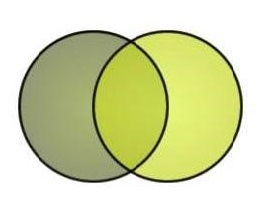# surface area

n=4

a: surface area ~
b: a balloon around the volume of air it contains

What:

The early Greek mathematicians knew the formulas for the circumference and area of a circle, and they knew the formulas for the surface area and the volume of a sphere, but no one noticed that the change in formula from circumference to area is the same as the change in formula from surface area to volume, as we show below. These two changes are similar because they both go from the formula for outer envelope to the formula for what is contained: the circumference is like a fence around a field, which is the area, the thing contained. The surface area is like a balloon around the volume of air it contains.

Useful?
Writer: Not Stated
LCC:
Where:
Date: Mar 3 2014 11:21 AM

a: surface area ~
b: concentration

What:

"Surface area is like concentration in that the greater numbers of
particles present means that a useful collision is more likely
(collision theory). Catalysts provide a surface area for reactions to
take place on and so also increase the chances of a reaction. "

Useful?
Writer: Not Stated
LCC:
Where:
Date: Mar 3 2014 11:23 AM

a: Surface Area to Volume Ratio ~
b: internal part

What:

"An object's Surface Area to Volume Ratio is like a way of describing how close every internal part of it is to its surface. It is worked out by dividing an object's surface area by its volume: A high S.A.:Vol Ratio shows that every part of an object is quite close to the edge. This means heat energy can get in and out quickly, ..

Useful?
Writer: Not Stated
LCC:
Where:
Date: Feb 11 2018 6:24 PM

a: Surface area inside the mitochondria ~
b: the table top

What:

"The surface area inside the mitochondria is like the table top where the reactions to break down food can take place. The more tabletop space you have, the more energy you can create. All around inside the mitochondria is a fluid called MATRIX."
The link contains a pretty good explanation for most parts of the mammalian cell.

Useful?
Writer: Not Stated
LCC: